# Linearized Equations in Cylindrical Coordinates

## Eulerian Formulation of Nonlinear Governing Equations

From our more detailed, accompanying discussion we pull the Eulerian representation of the set of principal governing equations written in cylindrical coordinates.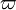$\varpi$ Component of Euler Equation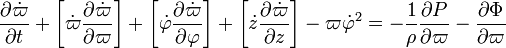$\frac{\partial \dot\varpi}{\partial t} + \biggl[ \dot\varpi \frac{\partial \dot\varpi}{\partial\varpi} \biggr] + \biggl[ \dot\varphi \frac{\partial \dot\varpi}{\partial\varphi} \biggr] + \biggl[ \dot{z} \frac{\partial \dot\varpi}{\partial z} \biggr] - \varpi {\dot\varphi}^2 = - \frac{1}{\rho}\frac{\partial P}{\partial\varpi} - \frac{\partial \Phi}{\partial\varpi}$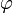$\varphi$ Component of Euler Equation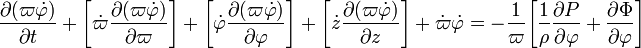$\frac{\partial (\varpi\dot\varphi)}{\partial t} + \biggl[ \dot\varpi \frac{\partial (\varpi\dot\varphi)}{\partial\varpi} \biggr] + \biggl[ \dot\varphi \frac{\partial (\varpi\dot\varphi)}{\partial\varphi} \biggr] + \biggl[ \dot{z} \frac{\partial (\varpi\dot\varphi)}{\partial z} \biggr] + \dot\varpi \dot\varphi = - \frac{1}{\varpi} \biggl[ \frac{1}{\rho}\frac{\partial P}{\partial \varphi} + \frac{\partial \Phi}{\partial \varphi} \biggr]$

z Component of Euler Equation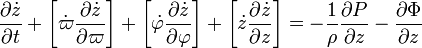$\frac{\partial \dot{z}}{\partial t} + \biggl[ \dot\varpi \frac{\partial \dot{z}}{\partial\varpi} \biggr] + \biggl[ \dot\varphi \frac{\partial \dot{z}}{\partial\varphi} \biggr] +\biggl[ \dot{z} \frac{\partial \dot{z}}{\partial z} \biggr] = - \frac{1}{\rho}\frac{\partial P}{\partial z} - \frac{\partial \Phi}{\partial z}$

Equation of Continuity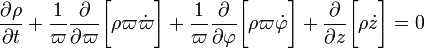$\frac{\partial\rho}{\partial t} + \frac{1}{\varpi} \frac{\partial}{\partial\varpi} \biggl[ \rho \varpi \dot\varpi \biggr] + \frac{1}{\varpi} \frac{\partial}{\partial \varphi} \biggl[ \rho \varpi \dot\varphi \biggr] + \frac{\partial}{\partial z} \biggl[ \rho \dot{z} \biggr] = 0$

These match, for example, equations (3.1) - (3.4) of Papaloizou & Pringle (1984, MNRAS, 208, 721-750), hereafter, PPI.

## Linearization

If we assume that the initial equilibrium configuration is axisymmetric with no radial or vertical velocity, the linearized equations become:

### Linearizing Radial Component of Euler Equation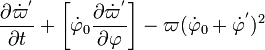$~\frac{\partial {\dot\varpi}^'}{\partial t} + \biggl[ {\dot\varphi}_0 \frac{\partial {\dot\varpi}^'}{\partial\varphi} \biggr] - \varpi ( { {\dot\varphi}_0 + {\dot\varphi}^'})^2$$~=$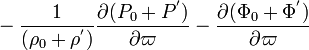$~- \frac{1}{(\rho_0 + \rho^')}\frac{\partial (P_0 + P^')}{\partial\varpi} - \frac{\partial (\Phi_0+\Phi^')}{\partial\varpi}$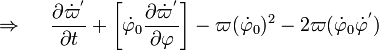$~\Rightarrow~~~~ \frac{\partial {\dot\varpi}^'}{\partial t} + \biggl[ {\dot\varphi}_0 \frac{\partial {\dot\varpi}^'}{\partial\varphi} \biggr] - \varpi ( {\dot\varphi}_0)^2 - 2\varpi ( {\dot\varphi}_0 {\dot\varphi}^')$$~=$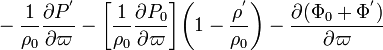$~ - \frac{1}{\rho_0}\frac{\partial P^'}{\partial\varpi} - \biggl[\frac{1}{\rho_0}\frac{\partial P_0 }{\partial\varpi}\biggr]\biggl(1 - \frac{\rho^'}{\rho_0} \biggr) - \frac{\partial (\Phi_0+\Phi^')}{\partial\varpi}$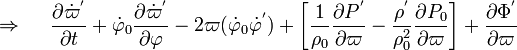$~\Rightarrow~~~~ \frac{\partial {\dot\varpi}^'}{\partial t} + {\dot\varphi}_0 \frac{\partial {\dot\varpi}^'}{\partial\varphi} - 2\varpi ( {\dot\varphi}_0 {\dot\varphi}^') + \biggl[ \frac{1}{\rho_0}\frac{\partial P^'}{\partial\varpi}- \frac{\rho^'}{\rho_0^2}\frac{\partial P_0 }{\partial\varpi}\biggr] + \frac{\partial \Phi^'}{\partial \varpi}$$~=$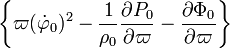$~\biggl\{ \varpi ( {\dot\varphi}_0)^2 - \frac{1}{\rho_0}\frac{\partial P_0 }{\partial\varpi} - \frac{\partial \Phi_0}{\partial\varpi} \biggr\}$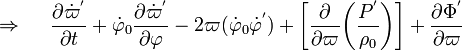$~\Rightarrow~~~~ \frac{\partial {\dot\varpi}^'}{\partial t} + {\dot\varphi}_0 \frac{\partial {\dot\varpi}^'}{\partial\varphi} - 2\varpi ( {\dot\varphi}_0 {\dot\varphi}^') + \biggl[ \frac{\partial}{\partial\varpi}\biggl( \frac{P^'}{\rho_0} \biggr) \biggr] + \frac{\partial \Phi^'}{\partial \varpi}$$~=$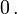$~0 \, .$

This last expression has been obtained by recognizing that, in the next-to-last expression: (1) The terms inside the curly braces on the right-hand side collectively provide a statement of equilibrium (in the radial-coordinate direction) in the initial, unperturbed configuration and therefore the terms sum to zero; and (2) the terms inside square brackets on the left-hand side can be rewritten in a more compact form because we have adopted a polytropic equation of state to build the unperturbed initial equilibrium configuration and are examining only adiabatic perturbations with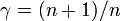$~\gamma = (n+1)/n$, in which case,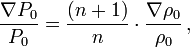$~\frac{\nabla P_0}{P_0} = \frac{(n+1)}{n} \cdot \frac{\nabla \rho_0}{\rho_0} \, ,$ and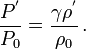$~\frac{P^'}{P_0} = \frac{\gamma \rho^'}{\rho_0} \, .$

### Linearizing Azimuthal Component of Euler Equation

Keeping in mind that the initial equilibrium configuration is axisymmetric — that is, equilibrium parameters exhibit no variation in the azimuthal direction — and, in addition,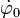$~\dot\varphi_0$ exhibits no variation in the vertical direction, we have,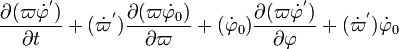$~\frac{\partial (\varpi {\dot\varphi}^')}{\partial t} + ( {\dot\varpi}^') \frac{\partial (\varpi\dot\varphi_0)}{\partial\varpi} + ( \dot\varphi_0)\frac{\partial (\varpi{\dot\varphi}^')}{\partial\varphi} + ( {\dot\varpi}^') {\dot\varphi_0}$$~=$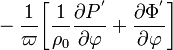$~- \frac{1}{\varpi} \biggl[ \frac{1}{\rho_0}\frac{\partial P^'}{\partial \varphi} + \frac{\partial \Phi^'}{\partial \varphi} \biggr]$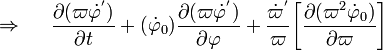$~\Rightarrow ~~~~\frac{\partial (\varpi {\dot\varphi}^')}{\partial t} + ( \dot\varphi_0)\frac{\partial (\varpi{\dot\varphi}^')}{\partial\varphi} + \frac{{\dot\varpi}^'}{\varpi}\biggl[ \frac{\partial (\varpi^2\dot\varphi_0)}{\partial\varpi} \biggr]$$~=$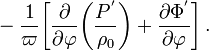$~- \frac{1}{\varpi} \biggl[ \frac{\partial }{\partial \varphi} \biggl(\frac{P^'}{\rho_0}\biggr)+ \frac{\partial \Phi^'}{\partial \varphi} \biggr] \, .$

### Linearizing Vertical Component of Euler Equation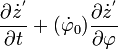$~ \frac{\partial {\dot{z}}^'}{\partial t} + (\dot\varphi_0) \frac{\partial {\dot{z}}^'}{\partial\varphi}$$~=$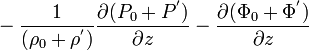$~ - \frac{1}{(\rho_0 + \rho^')}\frac{\partial (P_0 + P^')}{\partial z} - \frac{\partial (\Phi_0+\Phi^')}{\partial z}$$~=$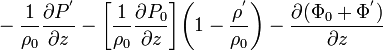$~ - \frac{1}{\rho_0}\frac{\partial P^'}{\partial z} - \biggl[\frac{1}{\rho_0}\frac{\partial P_0 }{\partial z}\biggr]\biggl(1 - \frac{\rho^'}{\rho_0} \biggr) - \frac{\partial (\Phi_0+\Phi^')}{\partial z}$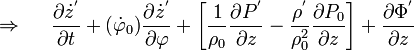$~\Rightarrow~~~~ \frac{\partial {\dot{z}}^'}{\partial t} + (\dot\varphi_0) \frac{\partial {\dot{z}}^'}{\partial\varphi} + \biggl[ \frac{1}{\rho_0}\frac{\partial P^'}{\partial z}- \frac{\rho^'}{\rho_0^2}\frac{\partial P_0 }{\partial z}\biggr] + \frac{\partial \Phi^'}{\partial z}$$~=$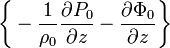$~\biggl\{ - \frac{1}{\rho_0}\frac{\partial P_0 }{\partial z} - \frac{\partial \Phi_0}{\partial z} \biggr\}$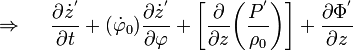$~\Rightarrow~~~~ \frac{\partial {\dot{z}}^'}{\partial t} + (\dot\varphi_0) \frac{\partial {\dot{z}}^'}{\partial\varphi} + \biggl[ \frac{\partial}{\partial z}\biggl( \frac{P^'}{\rho_0} \biggr) \biggr] + \frac{\partial \Phi^'}{\partial z}$$~=$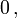$~0 \, ,$

where the logic followed in deriving the last expression from the next-to-last one is directly analogous to the logic used, above, in obtaining the final expression for the radial component of the linearized Euler equation.

### Linearizing Continuity Equation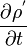$~\frac{\partial\rho^'}{\partial t}$$~=$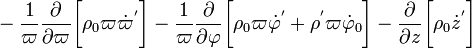$~ - \frac{1}{\varpi} \frac{\partial}{\partial\varpi} \biggl[ \rho_0 \varpi {\dot\varpi}^' \biggr] - \frac{1}{\varpi} \frac{\partial}{\partial \varphi} \biggl[ \rho_0 \varpi {\dot\varphi}^' + \rho^' \varpi {\dot\varphi}_0 \biggr] - \frac{\partial}{\partial z} \biggl[ \rho_0 {\dot{z}}^' \biggr]$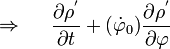$~\Rightarrow~~~~\frac{\partial\rho^'}{\partial t} + ( {\dot\varphi}_0 )\frac{\partial \rho^'}{\partial \varphi}$$~=$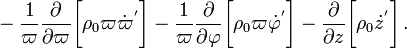$~ - \frac{1}{\varpi} \frac{\partial}{\partial\varpi} \biggl[ \rho_0 \varpi {\dot\varpi}^' \biggr] - \frac{1}{\varpi} \frac{\partial }{\partial \varphi} \biggl[ \rho_0 \varpi {\dot\varphi}^' \biggr] - \frac{\partial}{\partial z} \biggl[ \rho_0 {\dot{z}}^' \biggr] \, .$

### Summary

Set of Linearized Principal Governing Equations in Cylindrical Coordinates

 Continuity Equation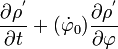$~\frac{\partial\rho^'}{\partial t} + ( {\dot\varphi}_0 )\frac{\partial \rho^'}{\partial \varphi}$$~=$$~ - \frac{1}{\varpi} \frac{\partial}{\partial\varpi} \biggl[ \rho_0 \varpi {\dot\varpi}^' \biggr] - \frac{1}{\varpi} \frac{\partial }{\partial \varphi} \biggl[ \rho_0 \varpi {\dot\varphi}^' \biggr] - \frac{\partial}{\partial z} \biggl[ \rho_0 {\dot{z}}^' \biggr] \, .$$\varpi$ Component of Euler Equation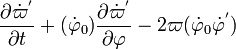$~ \frac{\partial {\dot\varpi}^'}{\partial t} + ( {\dot\varphi}_0 ) \frac{\partial {\dot\varpi}^'}{\partial\varphi} - 2\varpi ( {\dot\varphi}_0 {\dot\varphi}^')$$~=$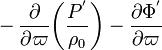$~ - \frac{\partial}{\partial\varpi}\biggl( \frac{P^'}{\rho_0} \biggr) - \frac{\partial \Phi^'}{\partial \varpi}$$\varphi$ Component of Euler Equation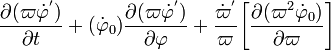$~\frac{\partial (\varpi {\dot\varphi}^')}{\partial t} + ( \dot\varphi_0)\frac{\partial (\varpi{\dot\varphi}^')}{\partial\varphi} + \frac{{\dot\varpi}^'}{\varpi}\biggl[ \frac{\partial (\varpi^2\dot\varphi_0)}{\partial\varpi} \biggr]$$~=$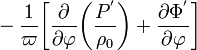$~- \frac{1}{\varpi} \biggl[ \frac{\partial }{\partial \varphi} \biggl(\frac{P^'}{\rho_0}\biggr)+ \frac{\partial \Phi^'}{\partial \varphi} \biggr]$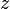$~z$ Component of Euler Equation$~ \frac{\partial {\dot{z}}^'}{\partial t} + (\dot\varphi_0) \frac{\partial {\dot{z}}^'}{\partial\varphi}$$~=$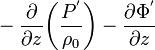$~ - \frac{\partial}{\partial z}\biggl( \frac{P^'}{\rho_0} \biggr) - \frac{\partial \Phi^'}{\partial z}$ Adiabatic Form of the 1st Law of Thermodynamics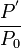$~\frac{P^'}{P_0}$$~=$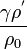$~ \frac{\gamma \rho^'}{\rho_0}$ Poisson Equation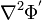$~\nabla^2 \Phi^'$$~=$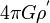$~ 4\pi G\rho^'$